## Intro

This vignette presents an example of using the onlineforecasting package for fitting a model and calculating forecasts as carried out by Bacher et al. (2013).

## Data

Data for the forecasting examples are taken from a data set collected in Sønderborg, Denmark. It comprises heat load measurements for sixteen houses, together with local climate observations and weather forecasts (NWPs). The houses are generally built in the sixties and seventies, with a floor plan in the range of 85 to 170 $$\mathrm{m^2}$$, and constructed in bricks. For each house only the total heat load, including both space heating and hot tap water heating, is available. The climate observations are measured at the local district heating plant within 10 kilometers from the houses. The NWPs are from the HIRLAM-S05 model and provided by the Danish Meteorological Institute. All times are in UTC and the time stamp for average values are set to the end of the time interval.

library(onlineforecast)

The Dbuilding data is included in the package. Its a data.list (see the vignette onlineforecasting.pdf):

class(Dbuilding)
##      "data.list"

Keep it in D and see the content:

D <- Dbuilding
names(D)
##      "I.obs"         "Ta"            "I"

The time:

head(D$t) ##  "2010-12-15 01:00:00 GMT" "2010-12-15 02:00:00 GMT" ##  "2010-12-15 03:00:00 GMT" "2010-12-15 04:00:00 GMT" ##  "2010-12-15 05:00:00 GMT" "2010-12-15 06:00:00 GMT" The observed heat load (in kW) of a house is kept in a data.frame: head(D$heatload)
##      5.92 5.85 5.85 5.88 5.85 5.83

The Numerical Weather Predictions (NWPs) of ambient temperature steps 1 to 9 hours ahead are:

head(D$Ta[ ,1:9]) ## k1 k2 k3 k4 k5 k6 k7 k8 k9 ## 1 -2.82 -3.20 -3.12 -3.09 -3.13 -3.16 -3.17 -3.09 -2.77 ## 2 -2.90 -3.12 -3.09 -3.13 -3.16 -3.17 -3.09 -2.77 -2.32 ## 3 -2.94 -3.09 -3.13 -3.16 -3.17 -3.09 -2.77 -2.32 -1.95 ## 4 -2.89 -3.11 -3.05 -3.11 -3.12 -2.81 -2.37 -2.01 -1.81 ## 5 -2.81 -3.05 -3.11 -3.12 -2.81 -2.37 -2.01 -1.81 -1.86 ## 6 -2.77 -3.11 -3.12 -2.81 -2.37 -2.01 -1.81 -1.86 -2.28 So at “2010-12-15 01:00:00 GMT” the latest available forecasts is the first row of Ta. We will add a y value into the data.list for simplicity and convention: D$y <- D$heatload Create a time of the day matrix for the forecast D$tday <- make_tday(D$t, 1:36) head(D$tday)
##       k1 k2 k3 k4 k5 k6 k7 k8 k9 k10 k11 k12 k13 k14 k15 k16 k17 k18 k19 k20 k21
##     1  2  3  4  5  6  7  8  9 10  11  12  13  14  15  16  17  18  19  20  21  22
##     2  3  4  5  6  7  8  9 10 11  12  13  14  15  16  17  18  19  20  21  22  23
##     3  4  5  6  7  8  9 10 11 12  13  14  15  16  17  18  19  20  21  22  23   0
##     4  5  6  7  8  9 10 11 12 13  14  15  16  17  18  19  20  21  22  23   0   1
##     5  6  7  8  9 10 11 12 13 14  15  16  17  18  19  20  21  22  23   0   1   2
##     6  7  8  9 10 11 12 13 14 15  16  17  18  19  20  21  22  23   0   1   2   3
##       k22 k23 k24 k25 k26 k27 k28 k29 k30 k31 k32 k33 k34 k35 k36
##     1  23   0   1   2   3   4   5   6   7   8   9  10  11  12  13
##     2   0   1   2   3   4   5   6   7   8   9  10  11  12  13  14
##     3   1   2   3   4   5   6   7   8   9  10  11  12  13  14  15
##     4   2   3   4   5   6   7   8   9  10  11  12  13  14  15  16
##     5   3   4   5   6   7   8   9  10  11  12  13  14  15  16  17
##     6   4   5   6   7   8   9  10  11  12  13  14  15  16  17  18

A time series plot, see “?plot_ts.data.list” (Note how the forecasts Ta are lagged to be synced with observations (i.e. then also with each other)):

plot_ts(D, c("^y","Ta"), kseq=c(1,12))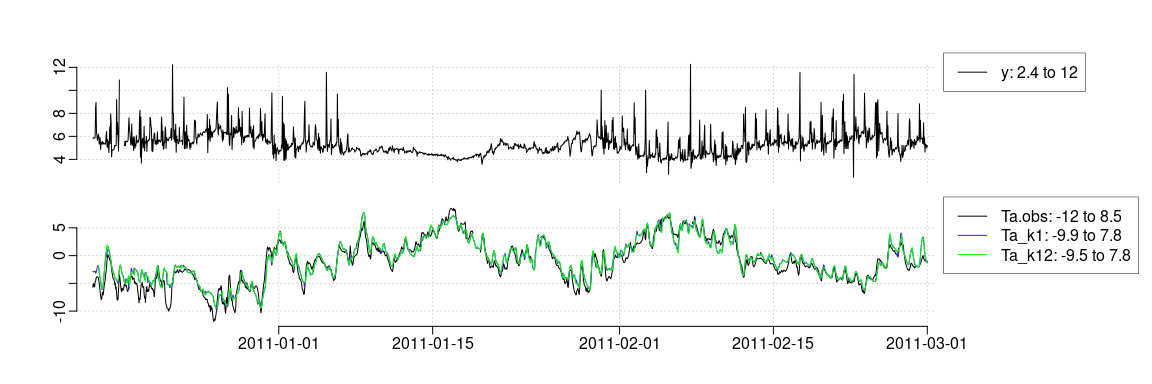A shorter period:

plot_ts(D, c("^y","Ta"), c("2010-12-15","2010-12-25"), kseq=c(1,12))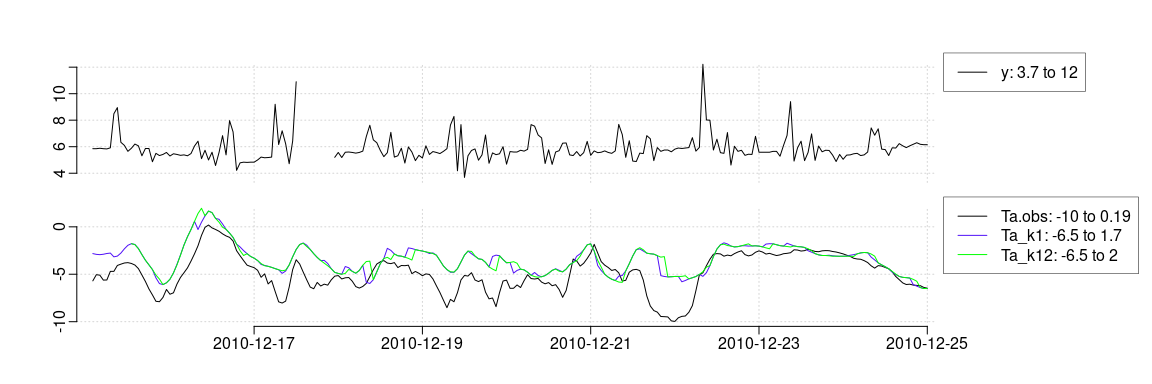Set the index of the training period and which period to evaluate (when fitting the points with scoreperiod==false are not included in the score evaluation):

Dtrain <- subset(D, c("2010-12-15", "2011-01-01"))
Dtrain$scoreperiod <- in_range("2010-12-20", Dtrain$t)

Define a model:

model <- forecastmodel$new() model$output = "y"
model$add_inputs(Ta = "lp(Ta, a1=0.9)", I = "lp(I, a1=0.7)", mu_tday = "fs(tday/24, nharmonics=10)", mu = "one()") model$add_regprm("rls_prm(lambda=0.9)")

Define the parameters to be optimized offline (their lower, init and upper bound):

model$add_prmbounds(Ta__a1 = c(0.8, 0.9, 0.9999), I__a1 = c(0.4, 0.8, 0.9999), lambda = c(0.9, 0.99, 0.9999)) Tune the parameters: first set the horizons to run and the: model$kseq <- c(1,18)
##?? Update here to remove caching and add that to another vignette
model$prm <- rls_optim(model, Dtrain)$par

Now fit with the optimized parameters on the entire period:

model$kseq <- 1:36 val <- rls_fit(model$prm, model, D, returnanalysis = TRUE)
##     ----------------
##     Ta__a1  I__a1 lambda
##      0.904  0.693  0.997

Plot the forecasts (Yhat adheres to the forecast matrix format and in plot_ts the forecasts are lagged k steps to sync with the observations):

D$Yhat <- val$Yhat
plot_ts(D, c("^y|^Y"), c("2011-01-01","2011-02-01"), kseq = c(1,18))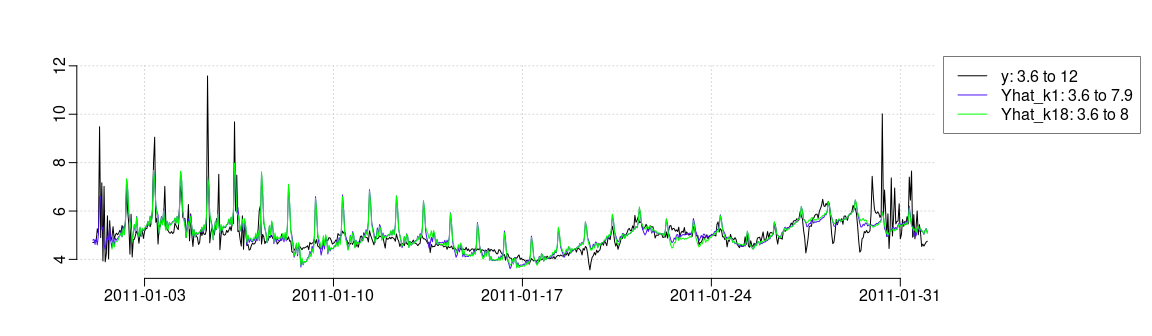Plot a forecast for a particular time point:

i <- 200
iseq <- i+model$kseq xplot <- data.frame(t=D$t[iseq], y=D$y[iseq], yhat=unlist(D$Yhat[i, ]))
plot_ts(xplot)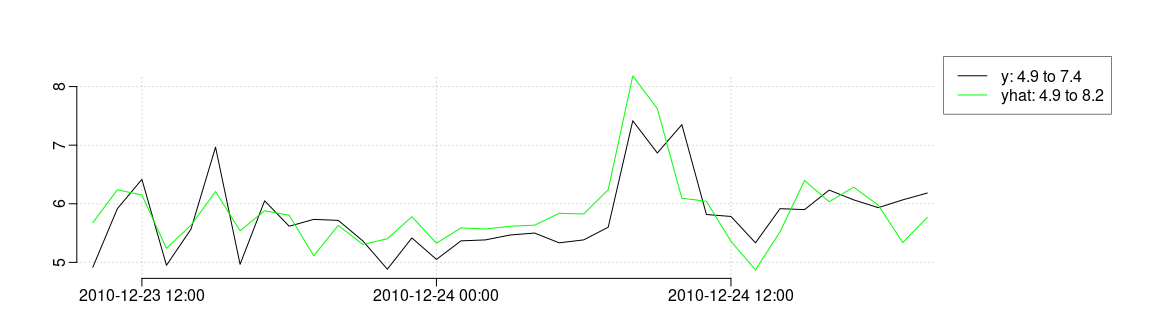### Recursive update and prediction

First fit on a period:

iseq <- which(in_range("2010-12-15",D$t,"2011-01-01")) Dfit <- subset(D, iseq) tmp <- rls_fit(model$prm, model, Dfit)
##     ----------------
##     Ta__a1  I__a1 lambda
##      0.904  0.693  0.997

Now the fits are saved in the model object (its an R6 object, hence passed by reference to the functions and can be changed inside the functions). A list of fits with an entry for each horizon is in Lfits, see the two first:

str(model$Lfits[1:2]) ## List of 2 ##$ k1:List of 4
##       ..$k : num 1 ## ..$ theta: num [1:23, 1] -0.17651 -0.00629 0.19557 -0.59015 -0.18158 ...
##       ..$P : num [1:23, 1:23] 1.12e-03 8.11e-05 1.85e-03 3.18e-03 -1.16e-03 ... ## .. ..- attr(*, "dimnames")=List of 2 ## .. .. ..$ : NULL
##       .. .. ..$: chr [1:23] "Ta" "I" "mu_tday.sin1" "mu_tday.cos1" ... ## ..$ yhat : num [1:36, 1] 6.44 6.62 6.49 6.86 6.14 ...
##      $k2:List of 4 ## ..$ k    : num 2
##       ..$theta: num [1:23, 1] -0.17939 -0.00466 0.24108 -0.48688 -0.22054 ... ## ..$ P    : num [1:23, 1:23] 1.11e-03 8.46e-06 4.08e-04 2.49e-05 -7.28e-05 ...
##       .. ..- attr(*, "dimnames")=List of 2
##       .. .. ..$: NULL ## .. .. ..$ : chr [1:23] "Ta" "I" "mu_tday.sin1" "mu_tday.cos1" ...
##       ..$yhat : num [1:36, 1] 6.53 6.42 6.79 6.06 5.85 ... Now new data arrives, take the point right after the fit period: (i <- iseq[length(iseq)] + 1) ##  409 Dnew <- subset(D, i) First we need to transform the new data (This must only be done once for each new data, since some transform functions, e.g. lp(), actually keep states, see the detailed vignette onlineforecasting.pdf): Dnew_transformed <- model$transform_data(Dnew)

Then we can update the parameters using the transformed data:

rls_update(model, Dnew_transformed, Dnew[[model$output]]) Calculate predictions using the new data and the updated fits (rls coefficient estimates are in knitr::inline_expr("model$Lfits[[k]]$theta)"): yhat <- rls_predict(model, Dnew_transformed) Plot to see that it fits the observations: iseq <- i + model$kseq
xplot <- data.frame(t=D$t[iseq], y=D$y[iseq], yhat=unlist(yhat))
plot_ts(xplot, mainouter=pst("Forecast available at time = ",D$t[i]))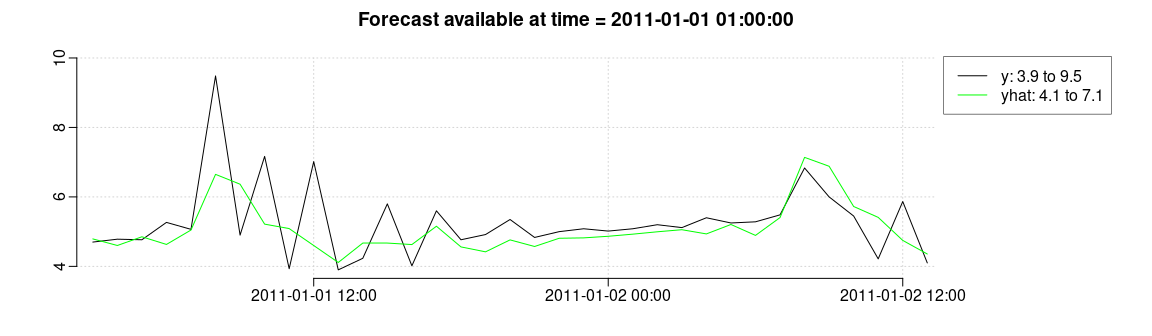Run this for a longer period to verify that the same forecasts are obtained (in one go vs. iteratively) First in one go: val <- rls_fit(model$prm, model, D, returnanalysis = TRUE)
##     ----------------
##     Ta__a1  I__a1 lambda
##      0.904  0.693  0.997
D$Yhat1 <- val$Yhat

and then iteratively (note i can take some time to run):

itrain <- which(in_range("2010-12-15",D$t,"2011-01-01")) itest <- which(in_range("2011-01-01",D$t,"2011-01-04"))
## ?? UPDATE to return invisible in rls_fit
tmp <- rls_fit(model$prm, model, subset(D, itrain)) ## ---------------- ## Ta__a1 I__a1 lambda ## 0.904 0.693 0.997 D$Yhat2 <- data.frame(matrix(NA, nrow(D$Yhat1), ncol(D$Yhat1)))
names(D$Yhat2) <- names(D$Yhat1)
for(i in itest){
Dnew <- subset(D, i)
Dnewtr <- model$transform_data(Dnew) rls_update(model, Dnewtr, Dnew[[model$output]])
D$Yhat2[i, ] <- as.numeric(rls_predict(model, Dnewtr)) } Compare to see the difference between the one step forecasts: D$Yhat1$k1[itest] - D$Yhat2\$k1[itest]
##        0.00e+00  0.00e+00  0.00e+00  0.00e+00  0.00e+00  0.00e+00  8.88e-16
##       -8.88e-16  0.00e+00  0.00e+00  0.00e+00  8.88e-16  0.00e+00  0.00e+00
##       0.00e+00  0.00e+00 -8.88e-16  0.00e+00  0.00e+00  0.00e+00  0.00e+00
##       0.00e+00  0.00e+00  8.88e-16  0.00e+00  0.00e+00  8.88e-16  0.00e+00
##       8.88e-16  0.00e+00 -8.88e-16  0.00e+00  0.00e+00  0.00e+00  0.00e+00
##       8.88e-16 -8.88e-16 -8.88e-16 -8.88e-16  0.00e+00  0.00e+00  0.00e+00
##       0.00e+00  0.00e+00  0.00e+00  0.00e+00  0.00e+00  0.00e+00  8.88e-16
##       8.88e-16  8.88e-16  0.00e+00  0.00e+00  0.00e+00  8.88e-16  0.00e+00
##       0.00e+00 -8.88e-16  0.00e+00  0.00e+00  0.00e+00  0.00e+00  0.00e+00
##       0.00e+00 -8.88e-16 -8.88e-16  0.00e+00  0.00e+00  0.00e+00  0.00e+00
##       0.00e+00  8.88e-16

## Literature

Bacher, Peder, Henrik Madsen, Henrik Aalborg Nielsen, and Bengt Perers. 2013. “Short-Term Heat Load Forecasting for Single Family Houses.” Energy and Buildings 65 (0): 101–12. https://doi.org/http://dx.doi.org/10.1016/j.enbuild.2013.04.022.